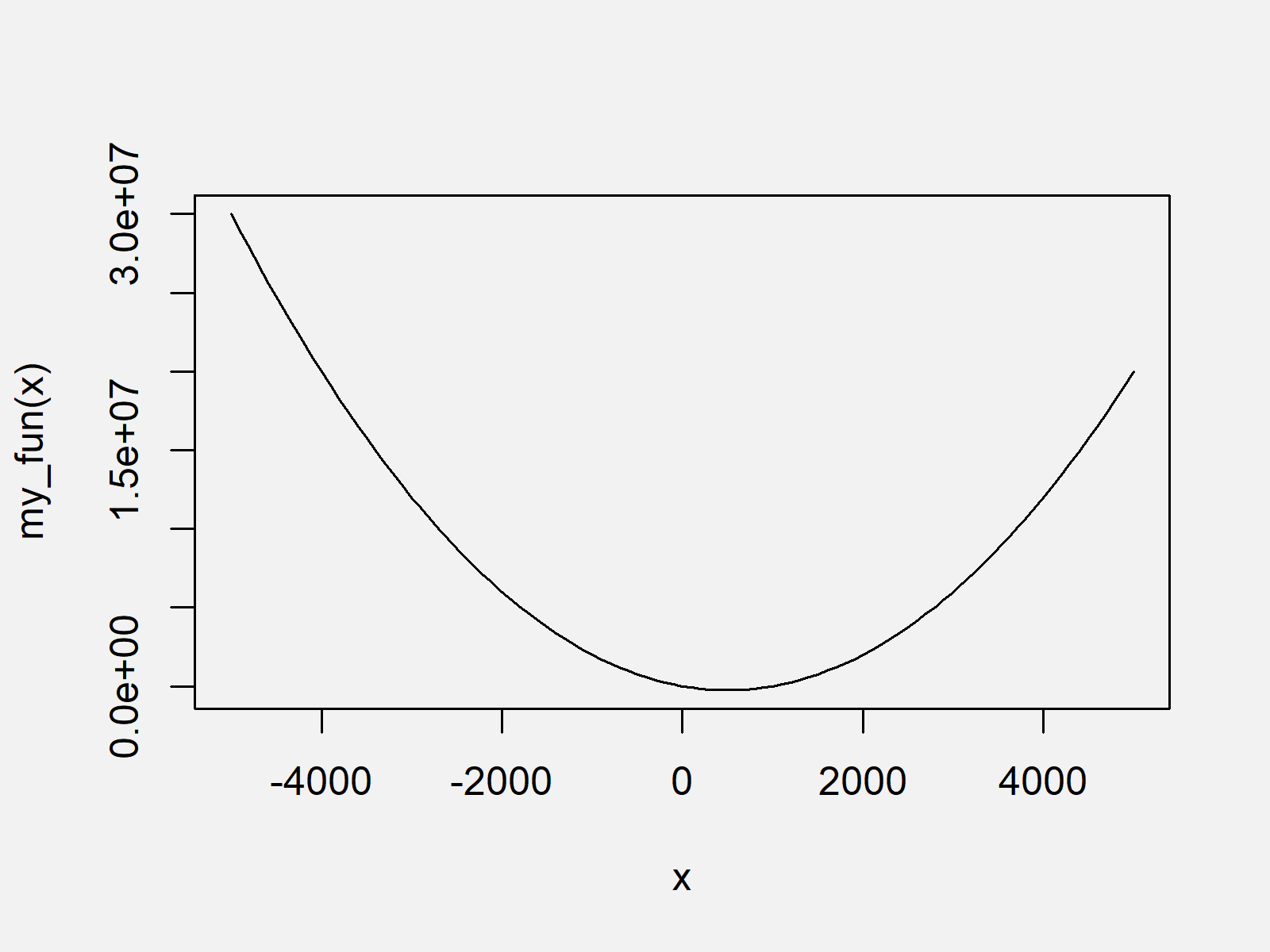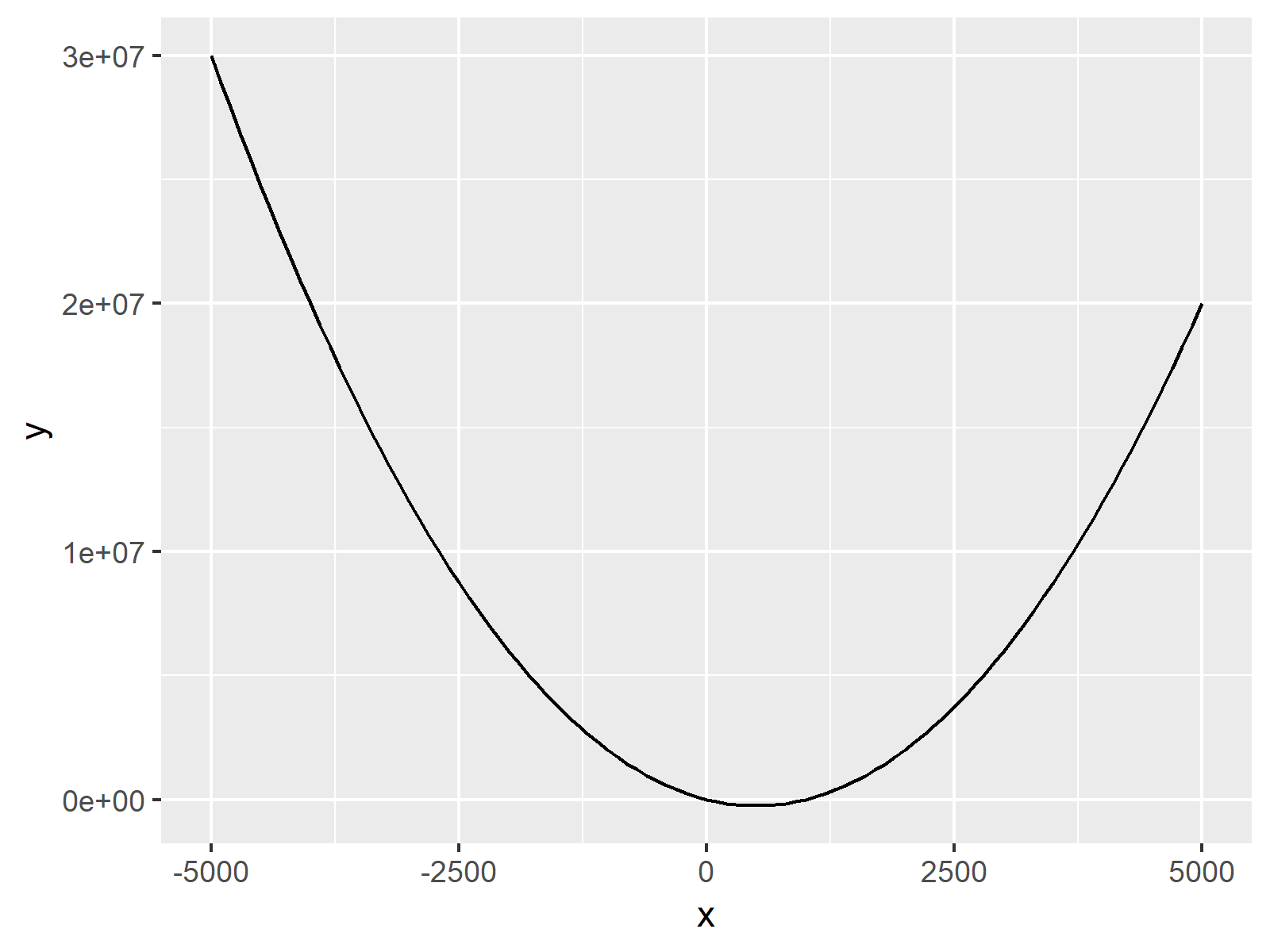# Draw Plot of Function Curve in R (2 Examples)

In this R tutorial you’ll learn how to create a plot showing the curve of a user-defined function.

The article will contain the following content:

So now the part you have been waiting for – the examples:

## Example Function

Let’s first create a user-defined function in R:

```my_fun <- function(x) {                        # Create own function
x^2 - x * 1000
}```

Next, I’ll show how to plot this function. So keep on reading!

## Example 1: Plotting Function Curve Using Base R

In Example 1, I’ll explain how to draw a function in a plot using the curve() command of the basic installation of R programming.

`curve(my_fun, from = - 5000, to = 5000)        # Apply curve`As you can see in Figure 1, we created a graphic showing our function with the previous R programming code.

## Example 2: Plotting Function Curve Using ggplot2 Package

Example 2 explains how to use the commands of the ggplot2 package to create a function plot. We first have to install and load the ggplot2 package:

```install.packages("ggplot2")                    # Install & load ggplot2 package
library("ggplot2")```

Furthermore, we have to create a data frame containing the range of input values between which we want to draw our function:

`data_fun <- data.frame(x = c(- 5000, 5000))    # Create data frame containing range`

Now, we can use the stat_function command of the ggplot2 package to draw our function:

```ggplot(data_fun, aes(x)) +                     # Draw ggplot2 plot
stat_function(fun = my_fun)```You can see the resulting graph in Figure 2. As you can see, it’s the same function as in Example 1, but this time in a different layout (which is prettier, if you ask me).

## Video, Further Resources & Summary

I have recently released a video on my YouTube channel, which illustrates the contents of this article. You can find the video below:

Please accept YouTube cookies to play this video. By accepting you will be accessing content from YouTube, a service provided by an external third party.If you accept this notice, your choice will be saved and the page will refresh.

In addition, you may want to have a look at some of the related R tutorials of Statistics Globe.

In summary: At this point you should have learned how to draw a manually specified function in the R programming language. Tell me about it in the comments, if you have further questions.

Subscribe to the Statistics Globe Newsletter